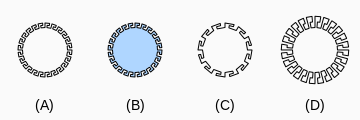OEDrawGreekKeyCircle¶

bool OEDrawGreekKeyCircle(OEDepict::OEImageBase &image,
const OEDepict::OEPen &pen, double patternAngle=15.0,
double patternWidthRatio=0.20)

Draws a circle with the OECircleStyle_GreekKey style.OEDrawGreekKeyCircle

image

The image on which the circle is drawn.

center

The center of the circle.

pen

The graphical properties of the circle.

See examples (A) and (B) in Figure: OEDrawGreekKeyCircle.

patternAngle

Specifies the angle (in degrees) between the patterns of the circle. The angle has to be in a range of $$[1.0^{\circ} - 90.0^{\circ}]$$

See example (C) in Figure: OEDrawGreekKeyCircle.

patternWidthRatio

Specifies the width of the pattern of the circle relative to its radius. The ratio has to be in a range of $$[0.01 - 1.00]$$.

See example (D) in Figure: OEDrawGreekKeyCircle.